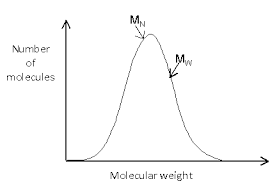## How to Calculate and Solve for Average Repeat Unit Molecular Weight for Co-Polymers | Polymer

The average repeat unit molecular weight for co-polymers is represented by the image below.To compute for average repeat unit molecular weight for co-polymers, one essential parameter is needed and the parameter is Numbers of co-polymers (n). By determining the number of co-polymers, four parameters is needed to get your answer so the parameters are Mole Fraction (f1), Molecular Weight (M1), Mole Fraction (f2) and Molecular Weight (M2).

The formula for calculating the average repeat unit molecular weight for co-polymers:

m’ = ΣfiMi

Let’s solve an example;
Find the average repeat unit molecular weight for co-polymers when the mole fraction(s) is 8,2 and the molecular weight(s) is 4,6.

This implies that;

m’ = ΣfiMi

Where:

m’ = Average Repeat Unit Molecular Weight for Co-Polymers
fi = Mole Fraction(s)
Mi = Molecular Weight(s)

m’ = (8)(4) + (2)(6)
m’ = (32) + (12)
m’ = 44

Therefore, the average repeat unit molecular weight for co-polymers is 44.